# RD Sharma solutions for Mathematics for Class 9 chapter 11 - Triangle and its Angles [Latest edition]

#### Chapters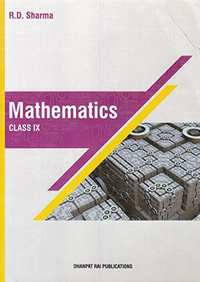## Solutions for Chapter 11: Triangle and its Angles

Below listed, you can find solutions for Chapter 11 of CBSE RD Sharma for Mathematics for Class 9.

Exercise 11.1Exercise 11.2Exercise 11.3Exercise 11.4
Exercise 11.1 [Page 10]

### RD Sharma solutions for Mathematics for Class 9 Chapter 11 Triangle and its Angles Exercise 11.1 [Page 10]

Exercise 11.1 | Q 1 | Page 10

In a ΔABC, if ∠A = 55°, ∠B = 40°, find ∠C.

Exercise 11.1 | Q 2 | Page 10

If the angles of a triangle are in the ratio 1: 2 : 3, determine three angles.

Exercise 11.1 | Q 3 | Page 10

The angles of a triangle are (x − 40)°, (x − 20)° and (1/2x-10)^@. find the value of x

Exercise 11.1 | Q 4 | Page 10

Two angles of a triangle are equal and the third angle is greater than each of those angles
by 30°. Determine all the angles of the triangle.

Exercise 11.1 | Q 5 | Page 10

If one angle of a triangle is equal to the sum of the other two, show that the triangle is a
right triangle.

Exercise 11.1 | Q 6.1 | Page 10

Can a triangle have two right angles? Justify your answer in case.

Exercise 11.1 | Q 6.2 | Page 10

Can a triangle have two obtuse angles?  Justify your answer in  case.

Exercise 11.1 | Q 6.3 | Page 10

Exercise 11.1 | Q 6.4 | Page 10

Can a triangle have  All angles more than 60°? Justify your answer in case.

Exercise 11.1 | Q 6.5 | Page 10

Can a triangle have All angles less than 60° Justify your answer in case.

Exercise 11.1 | Q 6.6 | Page 10

Can a triangle have All angles equal to 60°? Justify your answer in case.

Exercise 11.1 | Q 7 | Page 10

The angles of a triangle are arranged in ascending order of magnitude. If the difference
between two consecutive angles is 10°, find the three angles.

Exercise 11.1 | Q 8 | Page 10

ABC is a triangle in which ∠A — 72°, the internal bisectors of angles B and C meet in O.
Find the magnitude of ∠BOC.

Exercise 11.1 | Q 9 | Page 10

The bisectors of base angles of a triangle cannot enclose a right angle in any case.

Exercise 11.1 | Q 10 | Page 10

If the bisectors of the base angles of a triangle enclose an angle of 135°, prove that the triangle is a right triangle.

Exercise 11.1 | Q 11 | Page 10

In a ΔABC, ∠ABC = ∠ACB and the bisectors of ∠ABC and ∠ACB intersect at O such that ∠BOC = 120°. Show that ∠A = ∠B = ∠C = 60°.

Exercise 11.1 | Q 12 | Page 10

If each angle of a triangle is less than the sum of the other two, show that the triangle is acute angled.

Exercise 11.2 [Pages 19 - 22]

### RD Sharma solutions for Mathematics for Class 9 Chapter 11 Triangle and its Angles Exercise 11.2 [Pages 19 - 22]

Exercise 11.2 | Q 1 | Page 19

The exterior angles, obtained on producing the base of a triangle both way are 104° and 136°. Find all the angles of the triangle.

Exercise 11.2 | Q 2 | Page 19

In the given figure, the sides BC, CA and AB of a Δ ABC have been produced to D, E and F respectively. If ∠ACD = 105° and ∠EAF = 45°, find all the angles of the Δ ABC.

Exercise 11.2 | Q 3.1 | Page 20

Compute the value of x in the following figure: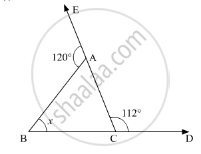Exercise 11.2 | Q 3.2 | Page 20

Compute the value of x in the following figure: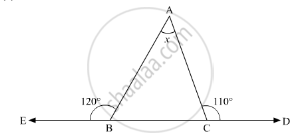Exercise 11.2 | Q 3.3 | Page 20

Compute the value of x in the following figure: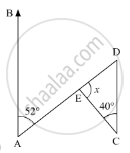Exercise 11.2 | Q 4 | Page 20

In the given figure, AC ⊥ CE and ∠A : ∠B : ∠C = 3 : 2 : 1, find the value of ∠ECD.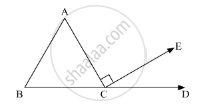Exercise 11.2 | Q 5 | Page 21

In the given figure, AB || DE. Find ∠ACD.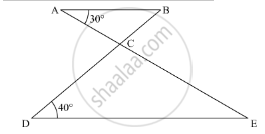Exercise 11.2 | Q 6.01 | Page 21

Is the following statement true and false :

Sum of the three angles of a triangle is 180 .

• True

• False

Exercise 11.2 | Q 6.02 | Page 21

Is the following statement true and false :

A triangle can have two right angles.

• True

• False

Exercise 11.2 | Q 6.03 | Page 21

Is the following statement true and false :

All the angles of a triangle can be less than 60°

• True

• False

Exercise 11.2 | Q 6.04 | Page 21

Is the following statement true and false :

All the angles of a triangle  can be greater than 60°.

• True

• False

Exercise 11.2 | Q 6.05 | Page 21

Is the following statement true and false :

All the angles of a triangle can be equal to 60°.

• True

• False

Exercise 11.2 | Q 6.06 | Page 21

Is the following statement true and false :

A triangle can have two obtuse angles.

• True

• False

Exercise 11.2 | Q 6.07 | Page 21

Is the following statement true and false :

A triangle can have at most one obtuse angles.

• Ture

• False

Exercise 11.2 | Q 6.08 | Page 21

Is the following statement true and false :

If one angle of a triangle is obtuse, then it cannot be a right angled triangle.

• Ture

• False

Exercise 11.2 | Q 6.09 | Page 21

Is the following statement true and false :

An exterior angle of a triangle is less than either of its interior opposite angles.

• Ture

• False

Exercise 11.2 | Q 6.1 | Page 21

Is the following statement true and false :

An exterior angle of a triangle is equal to the sum of the two interior opposite angles.

• Ture

• False

Exercise 11.2 | Q 6.11 | Page 21

Is the following statement true and false :

An exterior angle of a triangle is greater than the opposite interior angles.

• Ture

• False

Exercise 11.2 | Q 7.1 | Page 21

Fill in the blank to make the following statement true:

Sum of the angles of a triangle is ....

Exercise 11.2 | Q 7.2 | Page 21

Fill in the blank to make the following statement true:

An exterior angle of a triangle is equal to the two ....... opposite angles.

Exercise 11.2 | Q 7.3 | Page 21

Fill in the blank to make the following statement true:

An exterior angle of a triangle is always ......... than either of the interior opposite angles.

Exercise 11.2 | Q 7.4 | Page 21

Fill in the blank to make the following statement true:

A triangle cannot have more than ...... right angles.

Exercise 11.2 | Q 7.5 | Page 21

Fill in the blank to make the following statement true:

A triangles cannot have more than ......obtuse angles.

Exercise 11.2 | Q 8 | Page 21

In a Δ ABC, the internal bisectors of ∠B and ∠C meet at P and the external bisectors of ∠B and ∠C meet at Q, Prove that ∠BPC + ∠BQC = 180°.

Exercise 11.2 | Q 9 | Page 21

In the given figure, compute the value of x.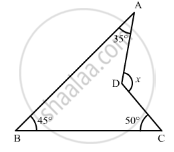Exercise 11.2 | Q 10 | Page 22

In the given figure, AB divides ∠DAC in the ratio 1 : 3 and AB = DB. Determine the value of x.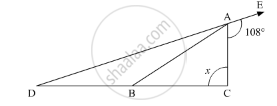Exercise 11.2 | Q 11 | Page 22

ABC is a triangle. The bisector of the exterior angle at B and the bisector of ∠C intersect each other at D. Prove that ∠D = $\frac{1}{2}$ ∠A.

Exercise 11.2 | Q 12 | Page 22

In the given figure, AM ⊥ BC and AN is the bisector of ∠A. If ∠B = 65° and ∠C = 33°, find ∠MAN.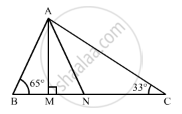Exercise 11.2 | Q 13 | Page 22

Exercise 11.2 | Q 14 | Page 22

In Δ ABC, BD⊥ AC and CE ⊥ AB. If BD and CE intersect at O, prove that ∠BOC = 180° − A.

Exercise 11.2 | Q 15 | Page 22

In the given figure, AE bisects ∠CAD and ∠B= ∠C. Prove that AE || BC.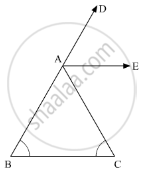Exercise 11.3 [Pages 23 - 24]

### RD Sharma solutions for Mathematics for Class 9 Chapter 11 Triangle and its Angles Exercise 11.3 [Pages 23 - 24]

Exercise 11.3 | Q 1 | Page 23

Define a triangle.

Exercise 11.3 | Q 2 | Page 23

Write the sum of the angles of an obtuse triangle.

Exercise 11.3 | Q 3 | Page 23

In Δ ABC, if u∠B = 60°, ∠C = 80° and the bisectors of angles ∠ABC and ∠ACB meet at a point O, then find the measure of ∠BOC.

Exercise 11.3 | Q 4 | Page 23

If the angles of a triangle are in the ratio 2 : 1 : 3, then find the measure of smallest angle.

Exercise 11.3 | Q 5 | Page 23

State exterior angle theorem.

Exercise 11.3 | Q 6 | Page 23

The sum of two angles of a triangle is equal to its third angle. Determine the measure of the third angle.

Exercise 11.3 | Q 7 | Page 23

In the given figure, if AB || CD, EF || BC, ∠BAC = 65° and ∠DHF = 35°, find ∠AGH.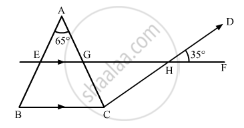Exercise 11.3 | Q 8 | Page 24

In the given figure, if AB || DE and BD || FG such that ∠FGH = 125° and ∠B = 55°, find x and y.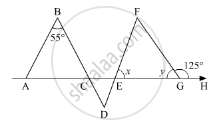Exercise 11.3 | Q 9 | Page 24

If the angles A, B and C of ΔABC satisfy the relation B − A = C − B, then find the measure of ∠B.

Exercise 11.3 | Q 10 | Page 24

In ΔABC, if bisectors of ∠ABC and ∠ACB intersect at O at angle of 120°, then find the measure of ∠A.

Exercise 11.3 | Q 11 | Page 24

If the side BC of ΔABC is produced on both sides, then write the difference between the sum of the exterior angles so formed and ∠A.

Exercise 11.3 | Q 12 | Page 24

In a triangle ABC, if AB =  AC and AB is produced to D such that BD =  BC, find ∠ACD: ∠ADC.

Exercise 11.3 | Q 13 | Page 24

In the given figure, side BC of ΔABC is produced to point D such that bisectors of ∠ABC and ∠ACD meet at a point E. If ∠BAC = 68°, find ∠BEC.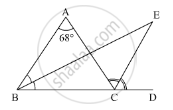Exercise 11.4 [Pages 25 - 29]

### RD Sharma solutions for Mathematics for Class 9 Chapter 11 Triangle and its Angles Exercise 11.4 [Pages 25 - 29]

Exercise 11.4 | Q 1 | Page 25

Mark the correct alternative in each of the following:
If all the three angles of a triangle are equal, then each one of them is equal to

•  90°

•  45°

•  60°

•  30°

Exercise 11.4 | Q 2 | Page 25

If two acute angles of a right triangle are equal, then each acute is equal to

• 30°

• 45°

• 60°

• 90°

Exercise 11.4 | Q 3 | Page 25

An exterior angle of a triangle is equal to 100° and two interior opposite angles are equal. Each of these angles is equal to

• 75°

• 80°

• 80°

• 40°

• 50°

Exercise 11.4 | Q 4 | Page 25

If one angle of a triangle is equal to the sum of the other two angles, then the triangle is

• an isosceles triangle

• an obtuse triangle

• an equilateral triangle

• a right triangle

Exercise 11.4 | Q 5 | Page 25

Side BC of a triangle ABC has been produced to a point D such that ∠ACD = 120°. If ∠B = $\frac{1}{2}$∠A is equal to

•  80°

•  75°

•  60°

•  90°

Exercise 11.4 | Q 6 | Page 25

In ΔABC, ∠B = ∠C and ray AX bisects the exterior angle ∠DAC. If ∠DAX = 70°, then ∠ACB =

• 35°

• 90°

• 70°

• 55°

Exercise 11.4 | Q 7 | Page 25

In a triangle, an exterior angle at a vertex is 95° and its one of the interior opposite angle is 55°, then the measure of the other interior angle is

•  55°

•  85°

•  40°

•  9.0°

Exercise 11.4 | Q 8 | Page 25

If the sides of a triangle are produced in order, then the sum of the three exterior angles so formed is

• 90°

• 180°

• 270°

• 360°

Exercise 11.4 | Q 9 | Page 25

In ΔABC, if ∠A = 100°, AD bisects ∠A and AD ⊥ BC. Then, ∠B =

• 50°

• 90°

• 40°

• 100°

Exercise 11.4 | Q 10 | Page 25

An exterior angle of a triangle is 108° and its interior opposite angles are in the ratio 4 : 5. The angles of the triangle are

• 48°, 60°, 72°

•  50°, 60°, 70°

• 52°, 56°, 72°

• 42°, 60°, 76°

Exercise 11.4 | Q 11 | Page 25

In a ΔABC, if ∠A = 60°, ∠B = 80° and the bisectors of ∠B and ∠C meet at O, then ∠BOC =

• 60°

• 120°

• 150°

• 30°

Exercise 11.4 | Q 12 | Page 25

Line segments AB and CD intersect at O such that AC || DB. If ∠CAB = 45° and ∠CDB = 55°, then ∠BOD =

•  100°

•  80°

•  90°

•  135°

Exercise 11.4 | Q 13 | Page 26

In the given figure, if EC || AB, ∠ECD = 70° and ∠BDO = 20°, then ∠OBD is

• 20°

• 50°

• 60°

• 70°

Exercise 11.4 | Q 14 | Page 26

In the given figure, x + y =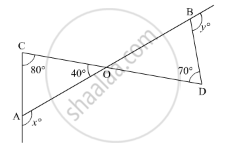• 270

• 230

•  210

• 190°

Exercise 11.4 | Q 15 | Page 26

If the measures of angles of a triangle are in the ratio of 3 : 4 : 5, what is the measure of the smallest angle of the triangle?

• 25°

• 30°

• 45°

• 60°

Exercise 11.4 | Q 16 | Page 26

In the given figure, if AB ⊥ BC. then x =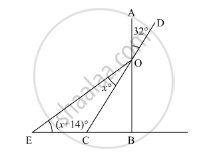• 18

• 22

• 25

• 32

Exercise 11.4 | Q 17 | Page 27

In the given figure, what is z in terms of x and y?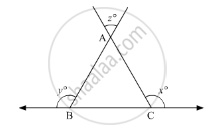• x + y + 180

•  x + y − 180

• 180° − (x + y)

•  x + y + 360°

Exercise 11.4 | Q 18 | Page 27

In the given figure, for which value of x is l1 || l2?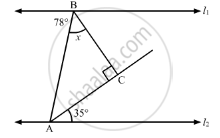• 37

• 43

• 45

• 47

Exercise 11.4 | Q 19 | Page 27

In the given figure, what is y in terms of x?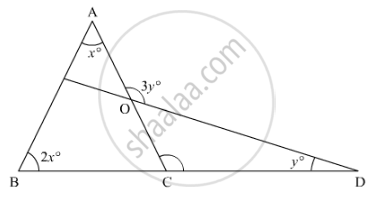• $\frac{3}{2}x$
• $\frac{4}{3}x$
• x
• $\frac{3}{4}x$

Exercise 11.4 | Q 20 | Page 27

In the given figure, what is the value of x?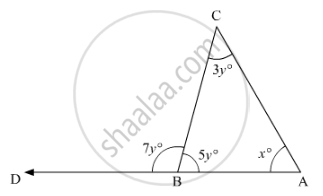• 35

• 45

• 50

• 60

Exercise 11.4 | Q 21 | Page 28

In the given figure, the value of x is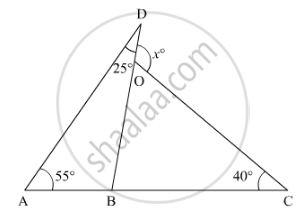• 65°

• 80°

• 95°

• 120°

Exercise 11.4 | Q 22 | Page 28

In the given figure, if BP || CQ and AC = BC, then the measure of x is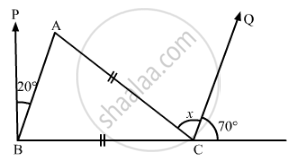•  20°

• 25°

•  30°

• 35°

Exercise 11.4 | Q 23 | Page 28

In the given figure, AB and CD are parallel lines and transversal EF intersects them at Pand Q respectively. If ∠APR = 25°, ∠RQC = 30° and ∠CQF = 65°, then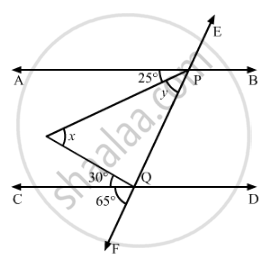• x = 55°, y = 40°

• x = 50°, y = 45°

• x = 60°, y = 35°

• x = 35°, y = 60°

Exercise 11.4 | Q 24 | Page 28

The base BC of triangle ABC is produced both ways and the measure of exterior angles formed are 94° and 126°. Then, ∠BAC =

• 94°

•  54°

•  40°

• 44°

Exercise 11.4 | Q 25 | Page 29

If the bisectors of the acute angles of a right triangle meet at O, then the angle at Obetween the two bisectors is

• 45°

•  95°

• 135°

• 90°

Exercise 11.4 | Q 26 | Page 29

The bisects of exterior angle at B and C of ΔABC meet at O. If ∠A = x°, then ∠BOC =

• $90^\circ + \frac{x^\circ }{2}$
• $90^\circ - \frac{x^\circ }{2}$

• $180^\circ + \frac{x^\circ }{2}$

• $180^\circ - \frac{x^\circ }{2}$

Exercise 11.4 | Q 27 | Page 29

In a ΔABC, ∠A = 50° and BC is produced to a point D. If the bisectors of ∠ABC and ∠ACDmeet at E, then ∠E =

• 25°

• 50°

• 100°

• 75°

Exercise 11.4 | Q 28 | Page 29

The side BC of ΔABC is produced to a point D. The bisector of ∠A meets side BC in L. If ∠ABC = 30° and ∠ACD = 115°, then ∠ALC =

•  85°

• $72\frac{1}{2}^\circ$
• 145°

• none of these

Exercise 11.4 | Q 29 | Page 29

In the given figure, if l1 || l2, the value of x is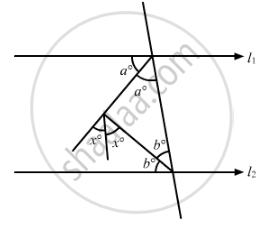• $22\frac{1}{2}$

• 30

• 45

• 60

Exercise 11.4 | Q 30 | Page 29

In ΔRST (See figure), what is the value of x?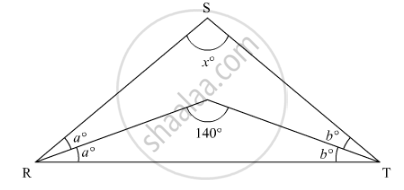• 40°

• 90°

• 80°

• 100°

## Solutions for Chapter 11: Triangle and its Angles

Exercise 11.1Exercise 11.2Exercise 11.3Exercise 11.4## RD Sharma solutions for Mathematics for Class 9 chapter 11 - Triangle and its Angles

Shaalaa.com has the CBSE Mathematics Mathematics for Class 9 CBSE solutions in a manner that help students grasp basic concepts better and faster. The detailed, step-by-step solutions will help you understand the concepts better and clarify any confusion. RD Sharma solutions for Mathematics Mathematics for Class 9 CBSE 11 (Triangle and its Angles) include all questions with answers and detailed explanations. This will clear students' doubts about questions and improve their application skills while preparing for board exams.

Further, we at Shaalaa.com provide such solutions so students can prepare for written exams. RD Sharma textbook solutions can be a core help for self-study and provide excellent self-help guidance for students.

Concepts covered in Mathematics for Class 9 chapter 11 Triangle and its Angles are Concept of Triangles - Sides, Angles, Vertices, Interior and Exterior of Triangle, Properties of a Triangle, Some More Criteria for Congruence of Triangles, Inequalities in a Triangle, Criteria for Congruence of Triangles, Congruence of Triangles.

Using RD Sharma Mathematics for Class 9 solutions Triangle and its Angles exercise by students is an easy way to prepare for the exams, as they involve solutions arranged chapter-wise and also page-wise. The questions involved in RD Sharma Solutions are essential questions that can be asked in the final exam. Maximum CBSE Mathematics for Class 9 students prefer RD Sharma Textbook Solutions to score more in exams.

Get the free view of Chapter 11, Triangle and its Angles Mathematics for Class 9 additional questions for Mathematics Mathematics for Class 9 CBSE, and you can use Shaalaa.com to keep it handy for your exam preparation.